Preliminary results as of Spring 2013
(FPCP 2013 conference)

 The global CKM fit: Inputs and Numerical results The global CKM fit in the large (ρ-bar,η-bar) plane Constraints on the angle α/ϕ2 from charmless B decays Constraints on the angle γ/ϕ3 from B decays to charm New physics in neutral-meson mixing Branching ratio of Bs→μ+ μ- The global CKM fit in the (|Vud|,|Vus|) plane The global CKM fit in the (|Vcd|,|Vcs|) plane Constraint from BR(B+→τ+ ν) The global CKM fit in the small (ρ-bar,η-bar) plane (zoom)

Inputs and numerical results:

 The results of the global CKM analysis include: Wolfenstein parameters, UT angles and sides, UTsangle and apex, CKM elements, input parameters, rare decay branching fractions.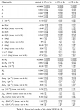Numerical Results

Constraints in the (ρ-bar,η-bar) plane. The red hashed region of the global combination corresponds to 68% CL.
 α, β, γconvention ϕ1, ϕ2, ϕ3convention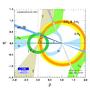eps png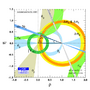eps png
Constraints in the (ρ-bar,η-bar) plane. The |Vub| constraint has been splitted in the two contributions: |Vub| from inclusive and exclusive semileptonic decays (plain dark green) and |Vub| from B+→τ+ ν (hashed green). The red hashed region of the global combination corresponds to 68% CL.
 α, β, γconvention ϕ1, ϕ2, ϕ3convention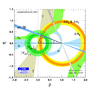eps png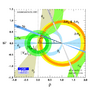eps png

Constraints on the angle α/ϕ2 from charmless B decays:

Constraints on α/ϕ2 from B→ρπ (BABAR, Belle and WA), with

α[WA,ρπ]=(54.1+7.7-10.3)° or (141.8+4.7-5.4

compared to the prediction from the global CKM fit (not including the α-related measurements)

α[fit]=(94.9+4.8-6.8

 α, β, γconvention ϕ1, ϕ2, ϕ3conventioneps png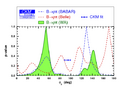eps png
Constraints on α/ϕ2 from the combination of B→ππ, ρπ, and ρρ for BABAR, Belle and WA, with

α[WA,all]=(85.4+4.0-3.8

compared to the prediction from the global CKM fit (not including the α-related measurements).
 α, β, γconvention ϕ1, ϕ2, ϕ3convention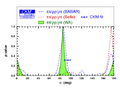eps png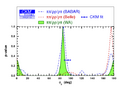eps png
Constraints on α/ϕ2 from B→ππ, ρπ and ρρ (WA) compared to the prediction from the global CKM fit (not including the α-related measurements).
 α, β, γconvention ϕ1, ϕ2, ϕ3convention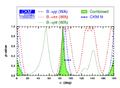eps png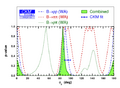eps png

The references of the observables from charged B → D(*)K(*) decays (GLW+ADS) and Dalitz analyses (GGSZ) from BaBar, Belle, LHCb are included in the summary of inputs and results file
We also use the inputs from charm (combining observables from charm mixing, CPV and ψ(3770) results):
• δD(Kπ) = (202.6+7.6-8.9
• δD(K3π) versus RK3π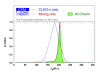eps png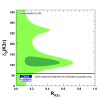eps png

Constraints on γ combining results from D(*)K(*) decays (GLW+ADS) and Dalitz analyses (GGSZ) from BaBar, Belle, LHCb and world average :
γ[combined] = (68.0+8.0-8.5)°,

compared to the prediction from the global CKM fit (not including these measurements): γ[fit] = (69.7+1.3-2.8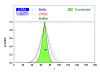eps png

Constraints on γ from world average D(*)K(*) decays (GLW+ADS) and Dalitz analyses (GGSZ) (methods shown separately):
• γ[BaBar] = (69 ± 17)°
• γ[Belle] = (68 ± 14)°
• γ[LHCb] = (69+11-13
• γ[combined] = (68.0+8.0-8.5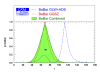eps png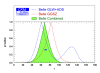eps png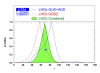eps png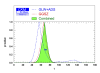eps png

Constraint on the ratio of interfering amplitudes rB of the decay B → DK from world average D(*)K(*) decays (GLW+ADS) and Dalitz analyses (GGSZ):
• rB(DK) = 0.0956+0.0062-0.0064
• rB(D*K) =0.121+0.018-0.019
• rB(DK*) = 0.140+0.044-0.047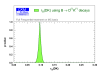eps pngeps png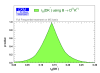eps png
Constraint on the strong phase between the interfering amplitudes of the decay B → DK from world average D(*)K(*) decays (GLW+ADS) and Dalitz analyses (GGSZ):
• δB(DK)=(114.8+9.0-9.7
• δB(D*K)=(-55+13-14)°
• δB(DK*)=(110+31-42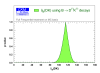eps png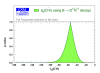eps png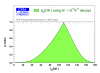eps png

Illustration of the correlation between the determination of γ and the ratio of interfering amplitudes rB (the strong phase difference δB) of the decay B → DK from world average D(*)K(*) decays (GLW+ADS) and Dalitz analyses (GGSZ).
 rB versus γ δB versus γ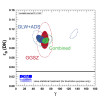eps png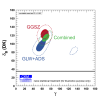eps png
Same using only Babar inputs.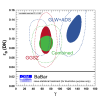eps png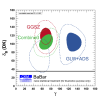eps png
Same using only Belle inputs.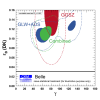eps png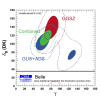eps png
Same using only LHCb inputs.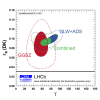eps png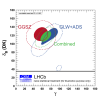eps png

Illustrations of the correlation between the determination of γ determined with the GLW+ADS observables and the strong phase difference δD for BaBar, Belle, LHCb and all experiments combined.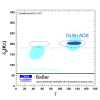eps png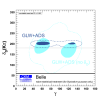eps png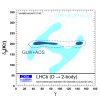eps png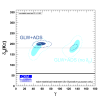eps png
Comparison of the strong phase δD(Kπ) obtained from charm inputs (green dotted), from GLW+ADS observables (blue) and from GLW+ADS+GGSZ observables (red)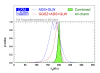eps pngIndividual constraints correspond to 68% CL (see: arXiv:1008.1593 [hep-ph] and arXiv:1203.0238 [hep-ph] for a detailed explanation of the hypotheses). Numerical inputs, results and confidence intervals are given in the detailed discussion of inputs and results.
 In scenario I we have introduced NP in M12q = M12SM,qΔq independently for Bd, Bs and K, corresponding to NP with arbitrary flavour structure. Constraints on New Physics in the (ReΔd,ImΔd) plane. A 1.5 σ deviation is obtained for the 2-dimensional SM hypothesis Δd = 1 (Re(Δd)=1, Im(Δd)=0).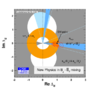eps png Constraints on New Physics in the (ReΔs,ImΔs) plane. A 0.0 σ deviation is obtained for the 2-dimensional SM hypothesis Δs = 1 (Re(Δs)=1, Im(Δs)=0). The pulls for the relevant observables are 0.2 σ for B->τν, 2.9 σ for φsJ/Ψ Φ and 3.4 σ for ASL, illustrating the difficulty to accomodate the last two results in this scenario.eps png
 Prediction for Br(Bs→μ+ μ-)=(3.63+0.21-0.34)x10-9. The blue (respectively red) curve represents the prediction removing the input from Δ ms (respectively fBs). The red curve is actually identical to the green one, indicating that the prediction is dominated by the indirect determination of fBs through the global fit (more specifically Δms), and not by its direct input.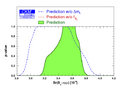eps png

 Constraints in the (|Vud|,|Vus|) plane. The indirect constraints (coming from b transitions) are related to |Vud| and |Vus| through unitarity. The red hashed region of the global combination corresponds to 68% CL.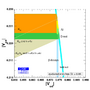eps png The accuracy recently reached by kaon leptonic decays K→eν and K→μν requires the inclusion of the full set of universal radiative corrections described in Marciano-Sirlin 1993. The agreement is then very good between the measurements and the prediction of the global fit without these observables.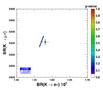eps png

 Constraints in the (|Vcd|,|Vcs|) plane. The indirect constraints (combing from b and s transitions) are related to |Vcd| and |Vcs| through unitarity. The direct constraints combine leptonic and semileptonic D and Ds decays as well as information from neutrino-nuclaon scattering and W → cs decays. The red hashed region of the global combination corresponds to 68% CL.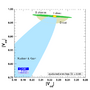eps png Constraints in the (|Vcd|,|Vcs|) plane where direct constraints involve only leptonic D and Ds decays with our inputs for lattice averages.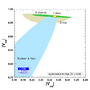eps png Constraints in the (|Vcd|,|Vcs|) plane where direct constraints involve only semileptonic D and Ds decays with our inputs for lattice averages.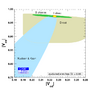eps png Constraints in the (|Vcd|,|Vcs|) plane where direct constraints involve only information from neutrino-nucleaon scattering and W→ cs decays (no lattice input).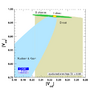eps png

 The recent update of BR(B→τν) from Belle has led to a decrease of the world average. The discrepancy in the CKM global fit between the world averages for sin2β and BR(B→τν) has thus been eased significantly. There is a specific correlation between the two quantities in the global fit which is best viewed in the (sin2β,BR(B→τν)) plane, regarding the prediction from the global fit without using these measurements. The cross corresponds to the experimental value with 1 sigma errors.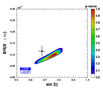eps png The constraint can also be seen from the point of view of lattice inputs, with the predictions on the decay constant fBd and fBd Sqrt[BBd]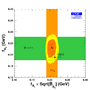eps png

Zoomed constraints in the (ρ-bar,η-bar) plane.The red hashed region of the global combination corresponds to 68% CL.
 α, β, γconvention ϕ1, ϕ2, ϕ3convention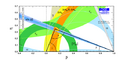eps png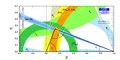eps png
Zoomed constraints in the (ρ-bar,η-bar) plane. The |Vub| constraint has been splitted in the two contributions: |Vub| from inclusive and exclusive semileptonic decays (plain dark green) and |Vub| from B+→τ+ ν (hashed green). The red hashed region of the global combination corresponds to 68% CL.
 α, β, γconvention ϕ1, ϕ2, ϕ3convention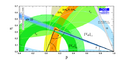eps png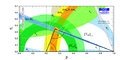eps png
Zoomed constraints in the (ρ-bar,η-bar) plane not including the angle measurements in the global fit.
 α, β, γconvention ϕ1, ϕ2, ϕ3convention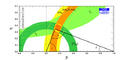eps png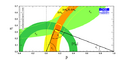eps png
Constraints in the (ρ-bar,η-bar) plane including only the angle measurements.
 α, β, γconvention ϕ1, ϕ2, ϕ3convention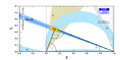eps png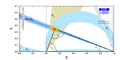eps png
Constraints from CP conserving quantities (|Vub / Vcb|, Δmd, (Δmd and Δms) and B+ →τ+ ν) in the (ρ-bar,η-bar) plane.
 α, β, γconvention ϕ1, ϕ2, ϕ3convention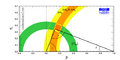eps png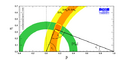eps png
Constraints from CP violating quantities (sin(2β), α, γ and εk) in the (ρ-bar,η-bar) plane.
 α, β, γconvention ϕ1, ϕ2, ϕ3conventioneps png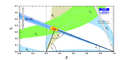eps png
Constraints from "Tree" quantities in the (ρ-bar,η-bar) plane (γ(DK) and α from the isospin analysis with the help of sin2β (charmonium), which gives another tree only γ measurement (the only assumption is that the ΔI=3/2 b-->d EW penguin amplitude is negligible)).
 α, β, γconvention ϕ1, ϕ2, ϕ3convention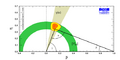eps png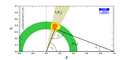eps png
Constraints from "Loop" quantities in the (ρ-bar,η-bar) plane.
 α, β, γconvention ϕ1, ϕ2, ϕ3convention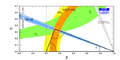eps png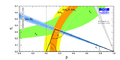eps png
Constraints in the (ρ-bar,η-bar) plane, not including the braching ratio of B+ → τ+ν in the global fit.
 α, β, γconvention ϕ1, ϕ2, ϕ3convention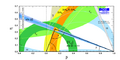eps png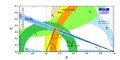eps png
Constraints in the (ρ-bar,η-bar) plane not including the measurement of sin2β in the global fit.
 α, β, γconvention ϕ1, ϕ2, ϕ3convention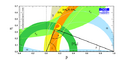eps png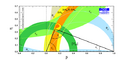eps png